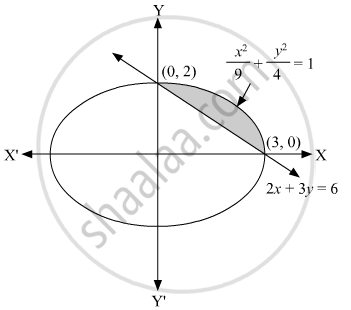# Using integration, find the area of the smaller region bounded by the ellipse "x"^2/9+"y"^2/4=1and the line "x"/3+"y"/2=1. - Mathematics

Sum

Using integration, find the area of the smaller region bounded by the ellipse "x"^2/9+"y"^2/4=1and the line "x"/3+"y"/2=1.

#### Solution

Given the equation of the ellipse is "x"^2/9+"y"^2/4=1

Let "y"_1=2/3sqrt(9-"x"^2) and

equation of the line is "x"/3+"y"/2=1

Let "y"_2=2/3(3-"x")

we have (3, 0) and (0, 2) as the points of intersection of ellipse and line.Therefore, the area of a smaller region, A =int_0^3("y"_1-"y"_2)"dx"

"A" =int_0^3[2/3sqrt(9-"x"^2)-2/3(3-"x")]"dx"

=int_0^3(2/3sqrt(9-"x"^2))"dx"-int_0^3[2/3(3-"x")]"dx"

=2/3["x"/2sqrt(9-"x"^2)+9/2sin^-1("x"/3)]_0^3-2/3(3"x"-"x"^2/2)_0^3

=2/3[(0+9/2xxpi/2)-0]-2/3(9-9/2-0)

=((3pi)/2-3) sq. unit

Concept: Area of the Region Bounded by a Curve and a Line
Is there an error in this question or solution?